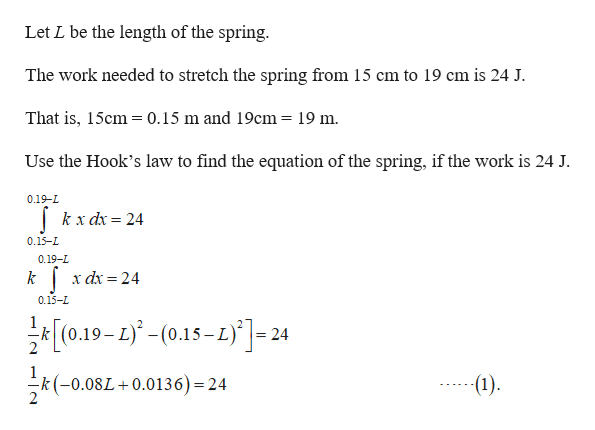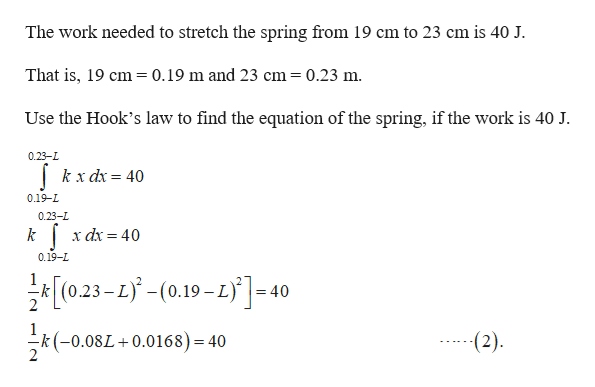# If 24 J of work are needed to stretch a spring from 15 cm to 19 cm and 40 J are needed to stretch it from 19 cm to 23 cm, what is the natural length of the spring?  I did the conversion to meters but in the end I didn't have the right answer, so I'm doing something wrong in my calculations.

Question
10 views

If 24 J of work are needed to stretch a spring from 15 cm to 19 cm and 40 J are needed to stretch it from 19 cm to 23 cm, what is the natural length of the spring?  I did the conversion to meters but in the end I didn't have the right answer, so I'm doing something wrong in my calculations.

check_circle

Step 1help_outlineImage TranscriptioncloseLet L be the length of the spring The work needed to stretch the spring from 15 cm to 19 cm is 24 J. That is, 15cm 0.15 m and 19cm = 19 m Use the Hook's law to find the equation of the spring, if the work is 24 J. 0.19-L kx d 24 0.15-L 0.19-L x dx 24 0.15-L [0.19-2-(0.15-1)]-24 -k(-0.08L+0.0136) = 24 (1) CN fullscreen
Step 2help_outlineImage TranscriptioncloseThe work needed to stretch the spring from 19 cm to 23 cm is 40 J. That is, 19 cm 0.19 m and 23 cm=0.23 m. Use the Hook's law to find the equation of the spring, if the work is 40 J. 0.23-L kx dx = 40 0.19-L 0.23-L x dx 40 = 0.19-L 023-(0.19-)40 2 (2) k (-0.08L0.0168) = 40 fullscreen

### Want to see the full answer?

See Solution

#### Want to see this answer and more?

Solutions are written by subject experts who are available 24/7. Questions are typically answered within 1 hour.*

See Solution
*Response times may vary by subject and question.
Tagged in

### Other